# 240 373 Image Processing Montri Karnjanadecha montricoe psu

• Slides: 22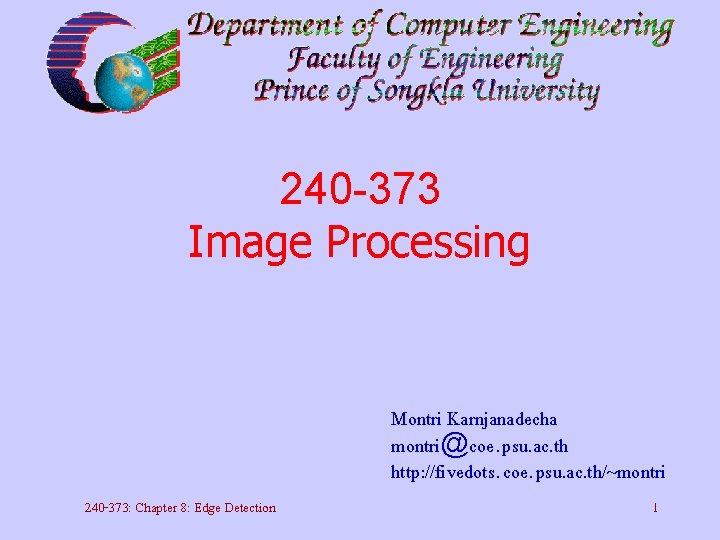240 -373 Image Processing Montri Karnjanadecha [email protected] psu. ac. th http: //fivedots. coe. psu. ac. th/~montri 240 -373: Chapter 8: Edge Detection 1Chapter 8 Edge Detection 240 -373: Chapter 8: Edge Detection 2Basic Edge Detection • Edge detection, ideally, identifies all the lines that outline the objects in an image. • Template for edge detection: Y_difference(x, y) = value(x, y) - value(x, y+1( This is equivalent to convolving with image with a 2 x 1 template 1 1240 -373: Chapter 8: Edge Detection 3Basic Edge Detection X_difference(x, y) = value(x, y) - value(x-1, y( use the template 1 1 - – Once X_difference and Y_difference are computed to create the single measurement of “gradient magnitude” (strength of the edge( 240 -373: Chapter 8: Edge Detection 4Basic Edge Detection – It is also useful to divide Y_difference by X_difference and identify a gradient direction (the angle of the edge between the regions( 240 -373: Chapter 8: Edge Detection 5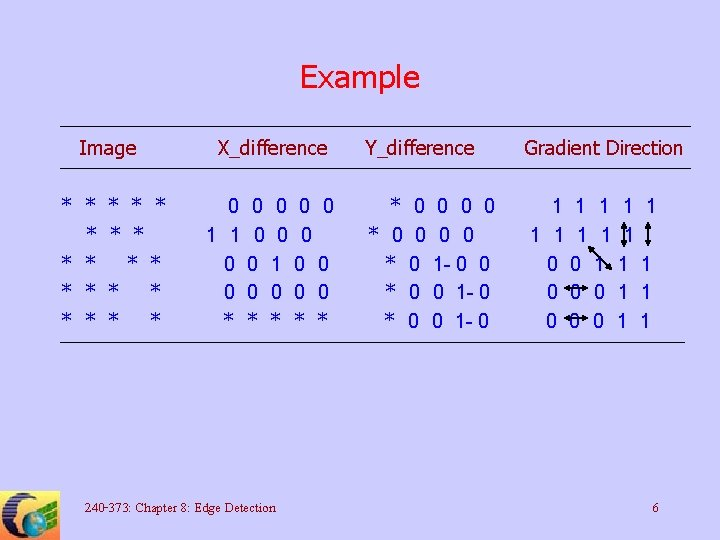Example Image * * * * X_difference * * 0 0 0 1 1 0 0 0 0 * * * 240 -373: Chapter 8: Edge Detection Y_difference Gradient Direction * 0 0 0 0 * 0 1 - 0 0 * 0 0 1 - 0 1 1 1 1 1 0 0 0 1 1 6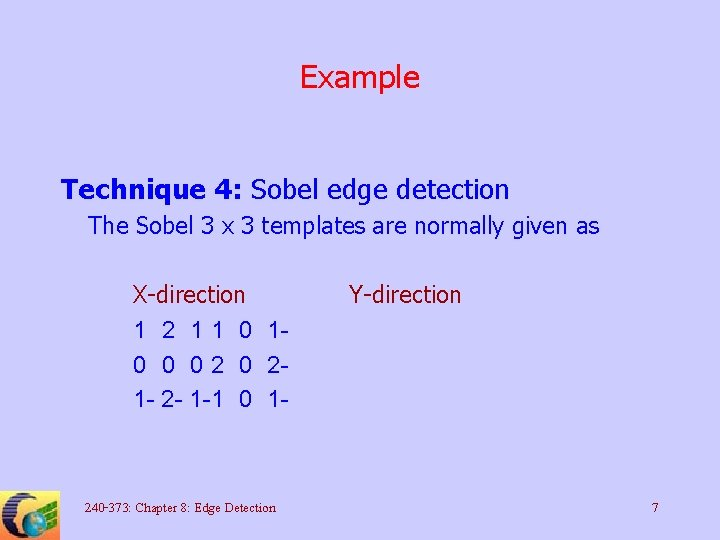Example Technique 4: Sobel edge detection The Sobel 3 x 3 templates are normally given as X-direction 1 2 1 1 0 10 0 0 21 - 2 - 1 -1 0 1 - 240 -373: Chapter 8: Edge Detection Y-direction 7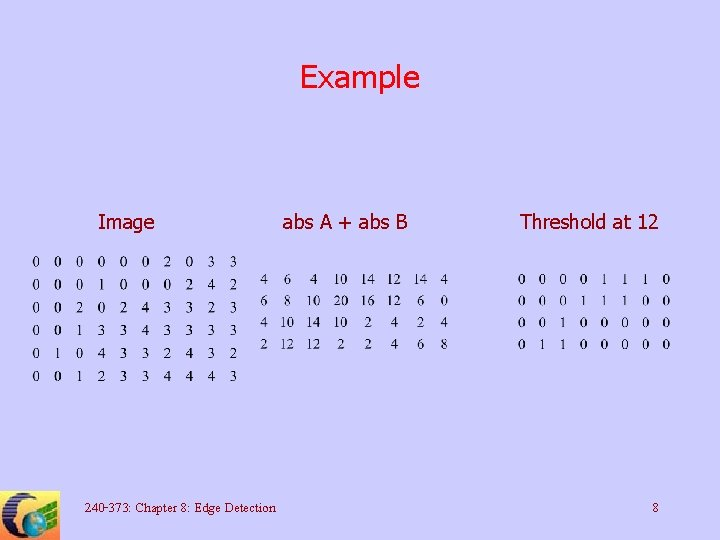Example Image 240 -373: Chapter 8: Edge Detection abs A + abs B Threshold at 12 8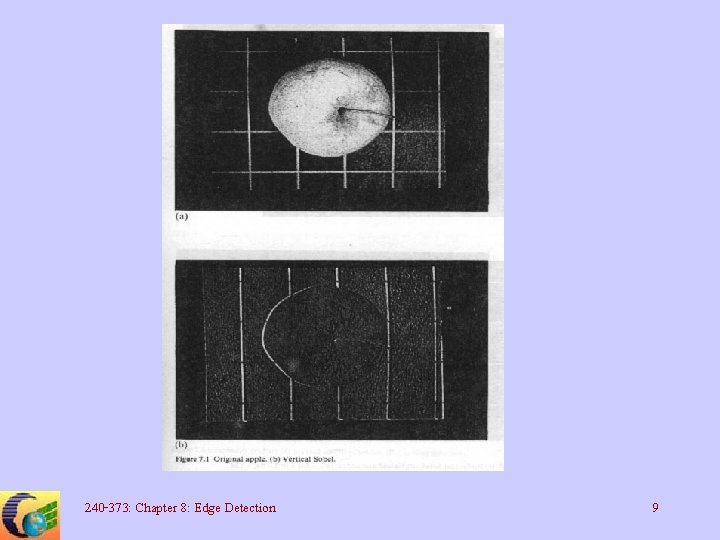240 -373: Chapter 8: Edge Detection 9240 -373: Chapter 8: Edge Detection 10240 -373: Chapter 8: Edge Detection 11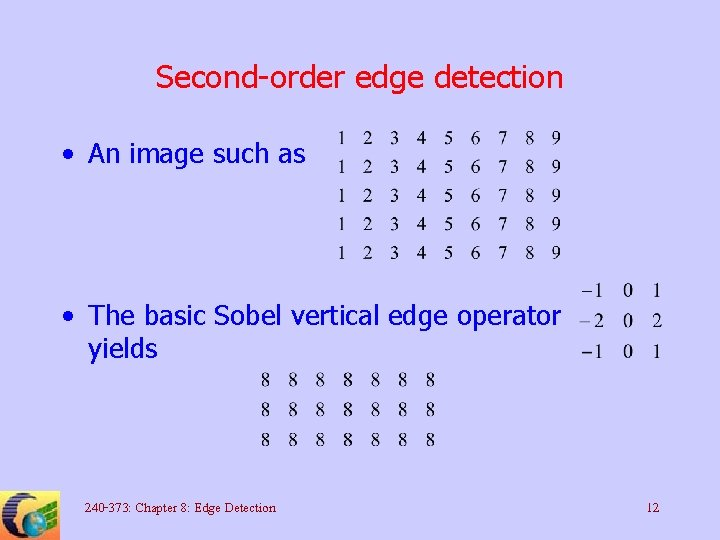Second-order edge detection • An image such as • The basic Sobel vertical edge operator yields 240 -373: Chapter 8: Edge Detection 12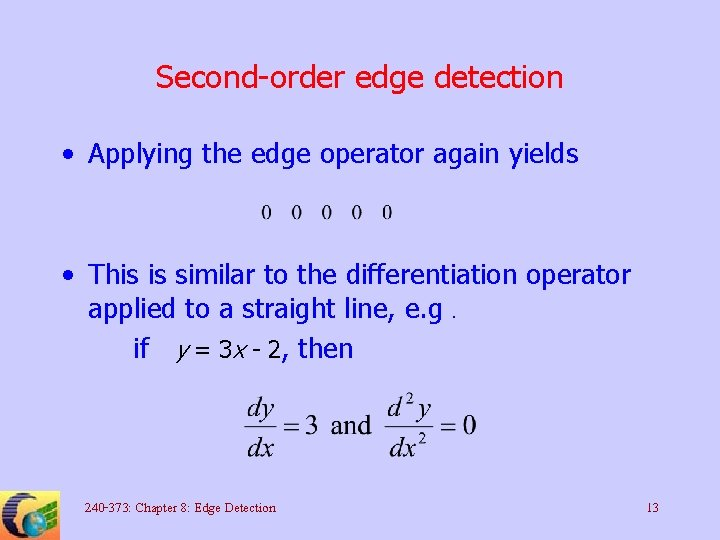Second-order edge detection • Applying the edge operator again yields • This is similar to the differentiation operator applied to a straight line, e. g. if y = 3 x - 2, then 240 -373: Chapter 8: Edge Detection 13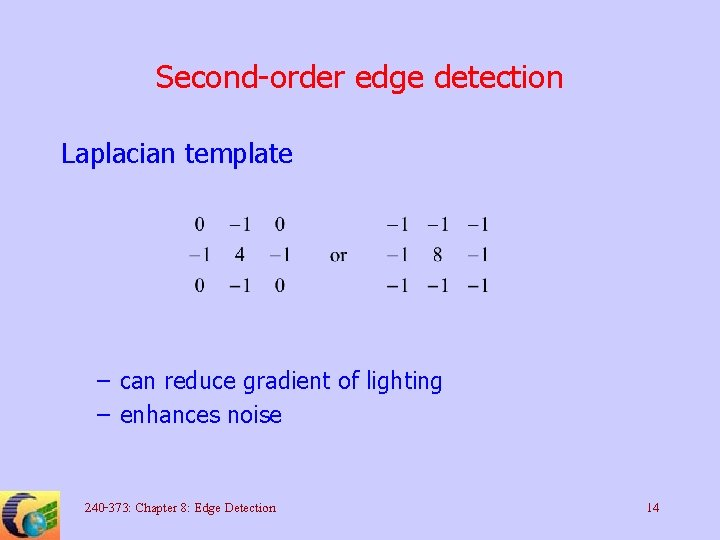Second-order edge detection Laplacian template – can reduce gradient of lighting – enhances noise 240 -373: Chapter 8: Edge Detection 14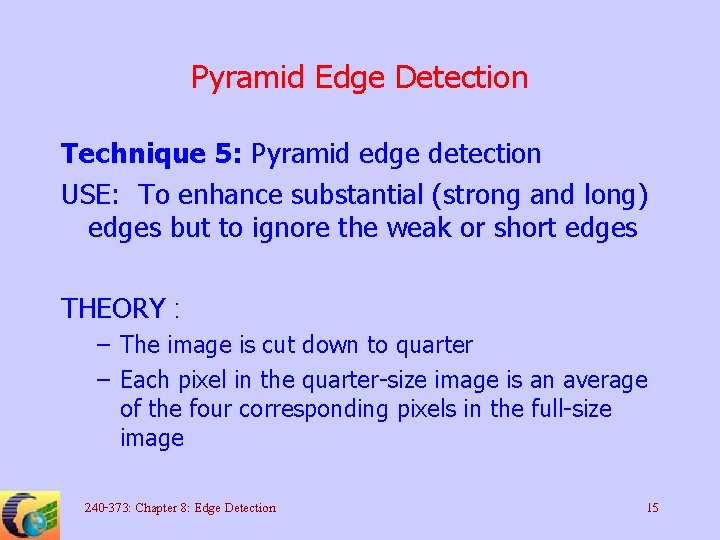Pyramid Edge Detection Technique 5: Pyramid edge detection USE: To enhance substantial (strong and long) edges but to ignore the weak or short edges THEORY : – The image is cut down to quarter – Each pixel in the quarter-size image is an average of the four corresponding pixels in the full-size image 240 -373: Chapter 8: Edge Detection 15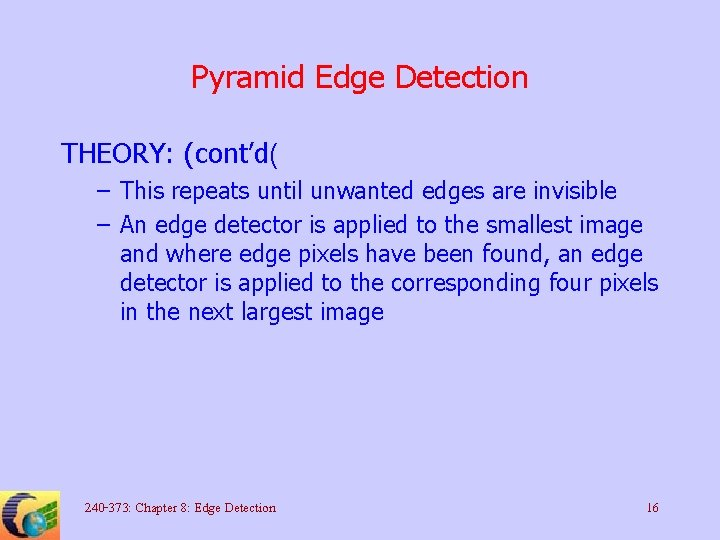Pyramid Edge Detection THEORY: (cont’d( – This repeats until unwanted edges are invisible – An edge detector is applied to the smallest image and where edge pixels have been found, an edge detector is applied to the corresponding four pixels in the next largest image 240 -373: Chapter 8: Edge Detection 16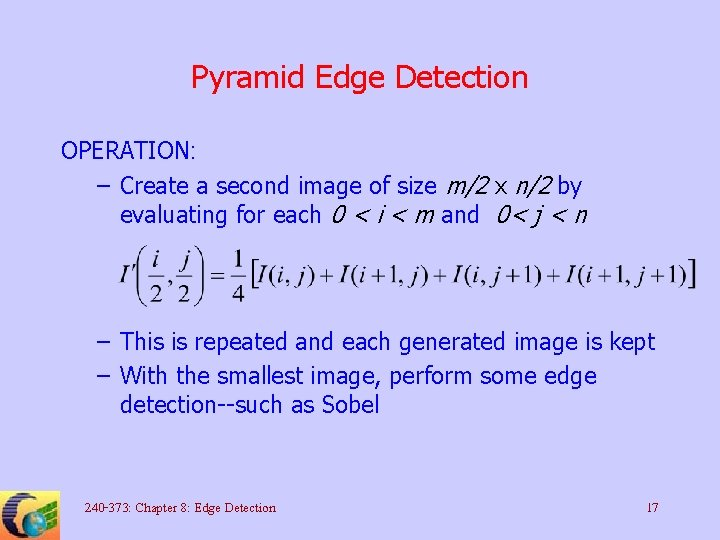Pyramid Edge Detection OPERATION: – Create a second image of size m/2 x n/2 by evaluating for each 0 < i < m and 0< j < n – This is repeated and each generated image is kept – With the smallest image, perform some edge detection--such as Sobel 240 -373: Chapter 8: Edge Detection 17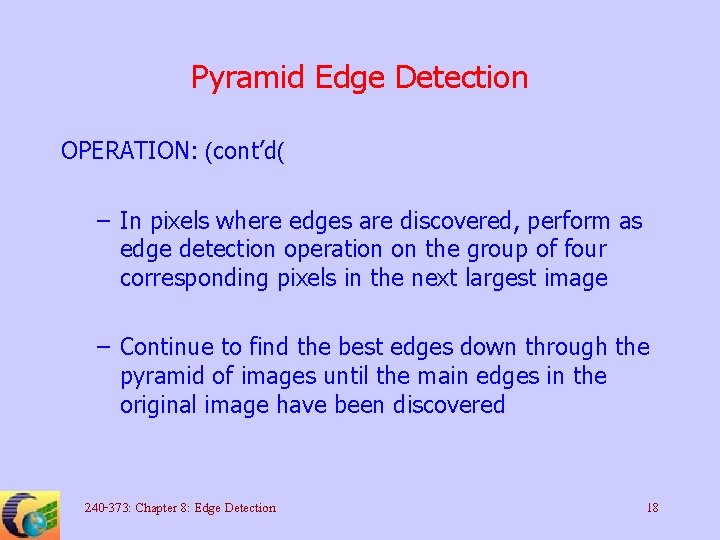Pyramid Edge Detection OPERATION: (cont’d( – In pixels where edges are discovered, perform as edge detection operation on the group of four corresponding pixels in the next largest image – Continue to find the best edges down through the pyramid of images until the main edges in the original image have been discovered 240 -373: Chapter 8: Edge Detection 18Edge Following Technique 7: Simple edge following USE: Knowing that a pixel is on an edge, the edge will be followed so that an objected is outlined OPERATION: – Suppose that a position on the edge has been identified, call it (x, y) and flag this position as used – Evaluate all the 3 x 3 Sobel gradient values centered on each of the eight pixels surrounding (x, y( 240 -373: Chapter 8: Edge Detection 19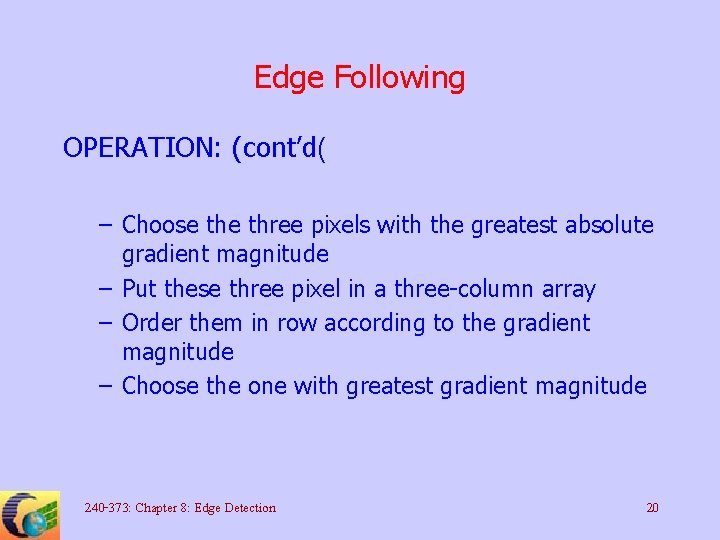Edge Following OPERATION: (cont’d( – Choose three pixels with the greatest absolute gradient magnitude – Put these three pixel in a three-column array – Order them in row according to the gradient magnitude – Choose the one with greatest gradient magnitude 240 -373: Chapter 8: Edge Detection 20Edge Following OPERATION: (cont’d( – Now this pixel will be one of the direction 0 -7 with respect to the pixel (x, y) given by the following map, where * is the position of pixel (x, y( 2 1 0 3 * 7 4 5 6 – Call the direction of travel d – Repeat the algorithm but look at only pixels in d, (d+1) mod 8, and (d-1) mod 8 directions 240 -373: Chapter 8: Edge Detection 21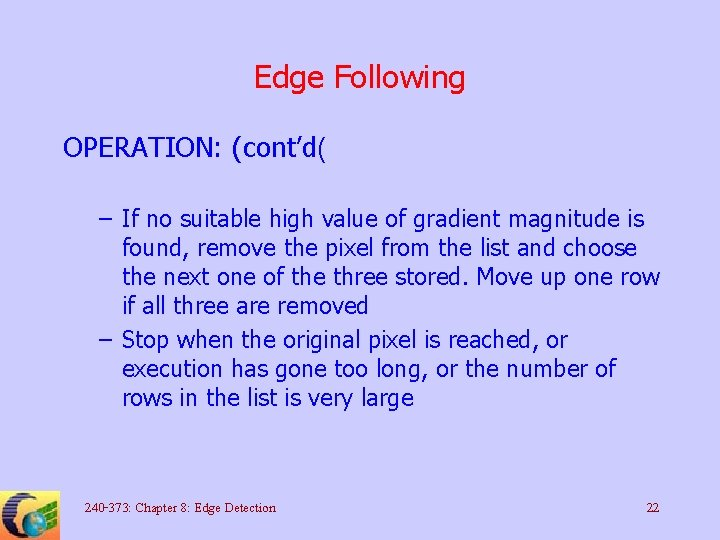Edge Following OPERATION: (cont’d( – If no suitable high value of gradient magnitude is found, remove the pixel from the list and choose the next one of the three stored. Move up one row if all three are removed – Stop when the original pixel is reached, or execution has gone too long, or the number of rows in the list is very large 240 -373: Chapter 8: Edge Detection 22